# Week 14 WAVES Light waves Sound waves Waves

• Slides: 32Week 14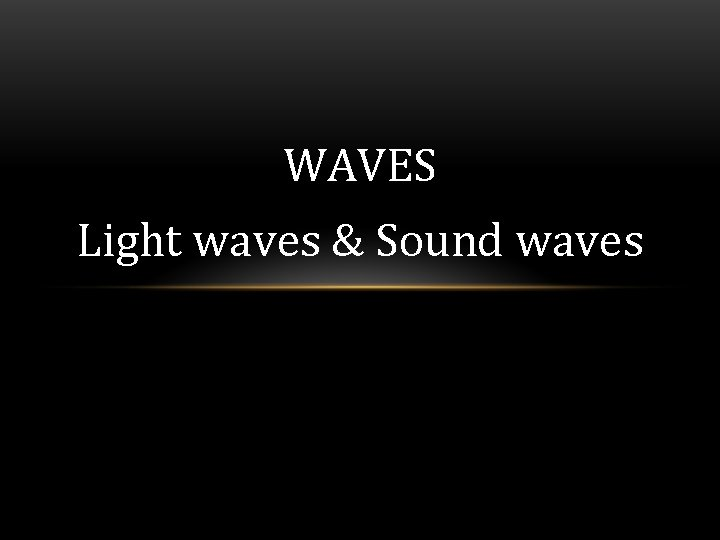WAVES Light waves & Sound waves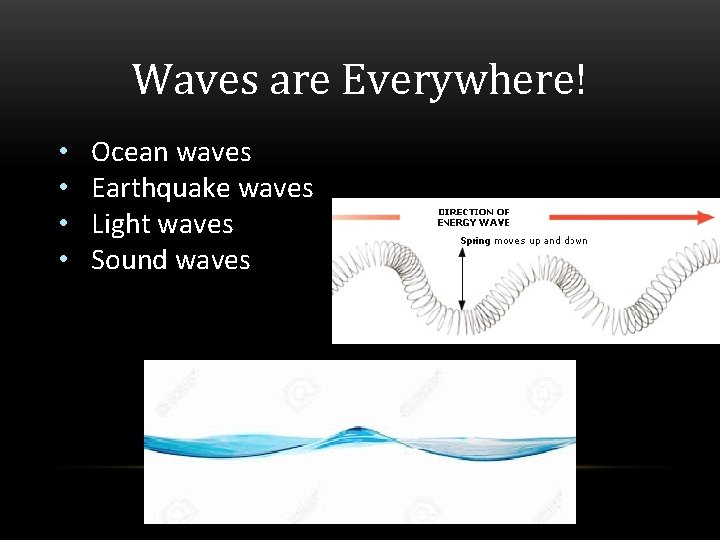Waves are Everywhere! • • Ocean waves Earthquake waves Light waves Sound wavesCreate a Wave 1. Fold construction paper in half (hotdog fold). Title top half of paper Transverse Wave. Title bottom half of paper Relationship between Frequency and Wavelength 2. At the top - create a transverse wave with 2 crest and 2 troughs using yarn. Label the following parts of the wave: amplitude, wavelength, rest position, crest and trough. The wave should start from the rest position. 3. At the bottom - create a wave with high frequency followed by low frequency. Label each section of the wave. Then explain how frequency and wavelength are related.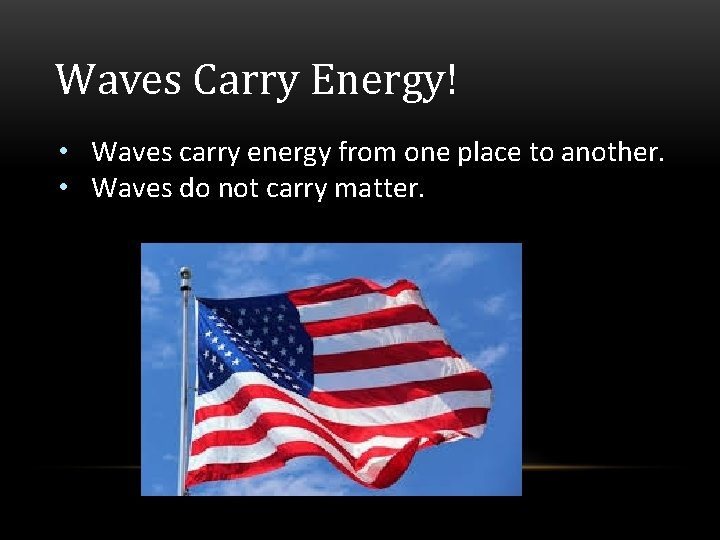Waves Carry Energy! • Waves carry energy from one place to another. • Waves do not carry matter.WAVE: (Definition) • A simple wave has a repeating pattern with a specific wavelength, frequency, and amplitude. • Definition: A disturbance that carries energy from one place to another.PARTS OF A WAVE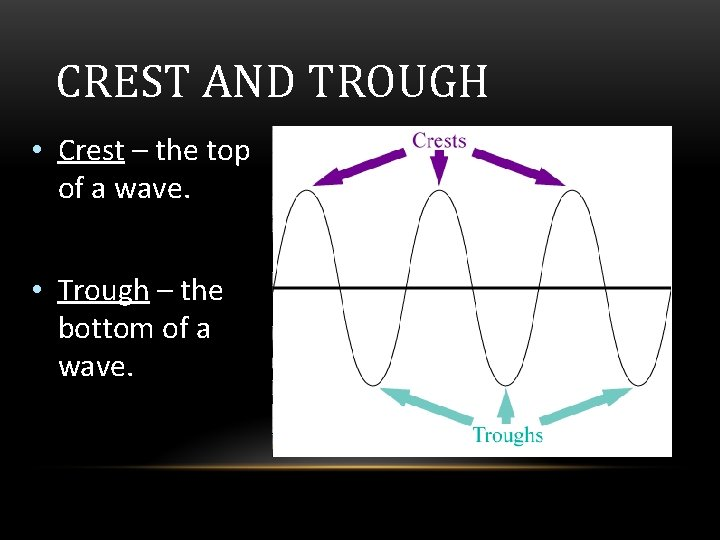CREST AND TROUGH • Crest – the top of a wave. • Trough – the bottom of a wave.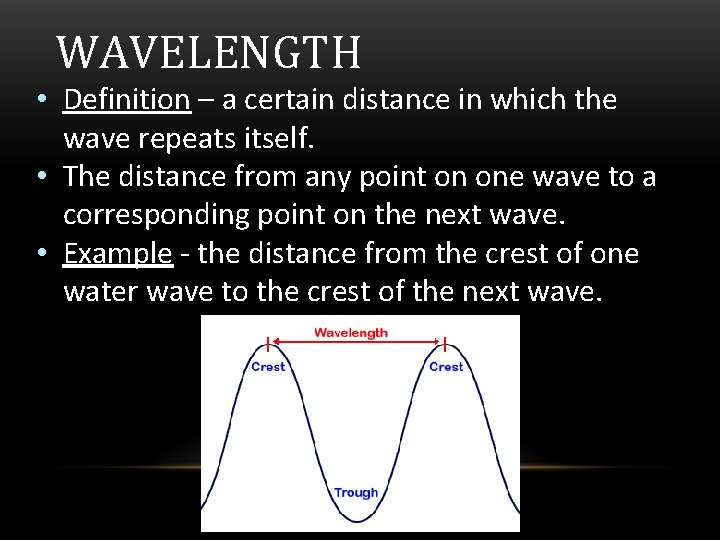WAVELENGTH • Definition – a certain distance in which the wave repeats itself. • The distance from any point on one wave to a corresponding point on the next wave. • Example - the distance from the crest of one water wave to the crest of the next wave.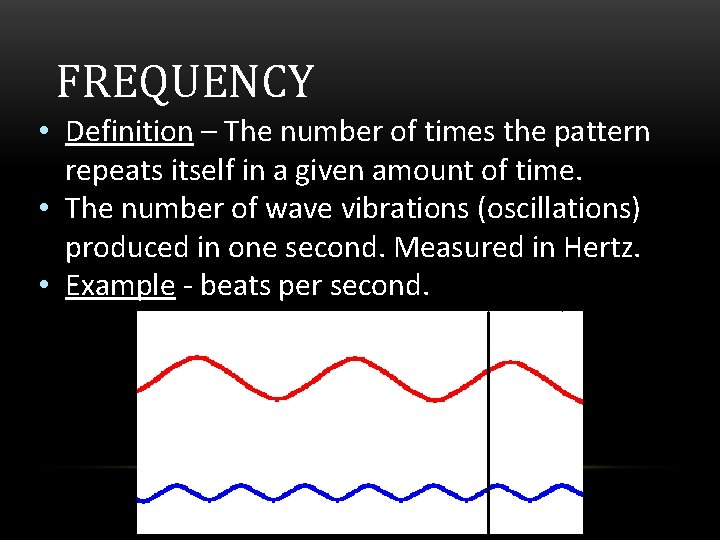FREQUENCY • Definition – The number of times the pattern repeats itself in a given amount of time. • The number of wave vibrations (oscillations) produced in one second. Measured in Hertz. • Example - beats per second.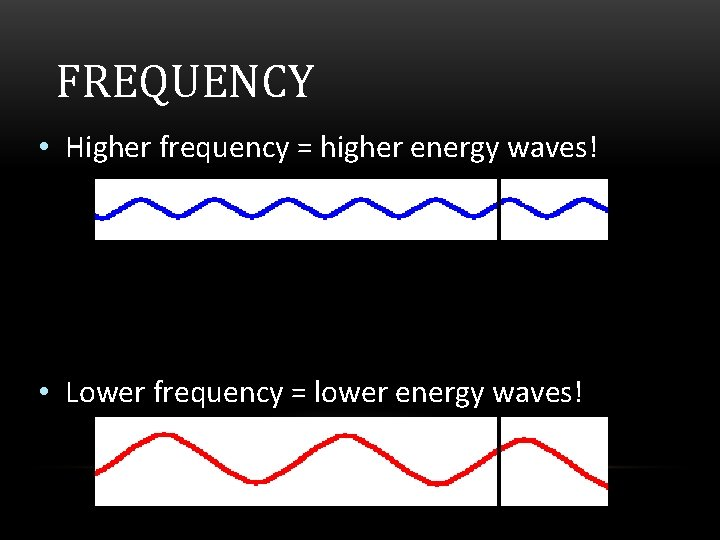FREQUENCY • Higher frequency = higher energy waves! • Lower frequency = lower energy waves!FREQUENCY & WAVELENGTH • Frequency and wavelength are related!!! • Low frequency = Long wavelength LOW Energy • High frequency = Short wavelength HIGH EnergyAMPLITUDE • The maximum height or depth of a wave. • The total distance a wave moves (oscillates) from its resting position. • Examples: • Sound waves get LOUDER. • Light waves get BRIGHTER.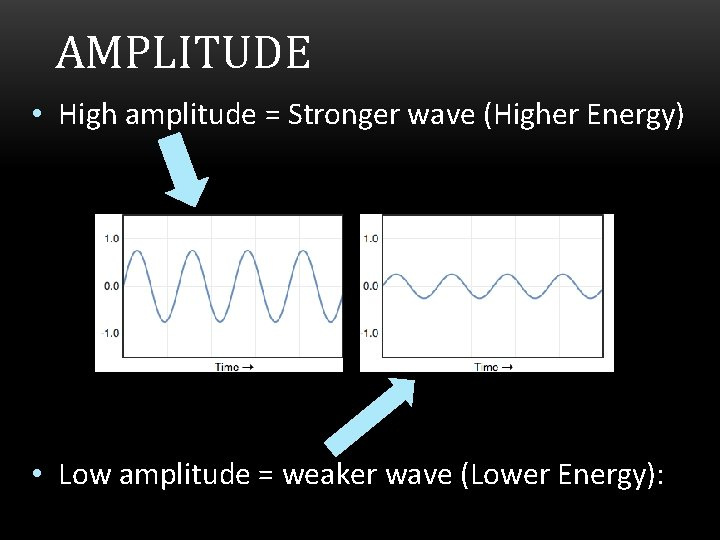AMPLITUDE • High amplitude = Stronger wave (Higher Energy) • Low amplitude = weaker wave (Lower Energy):PART 2: TWO TYPES OF WAVES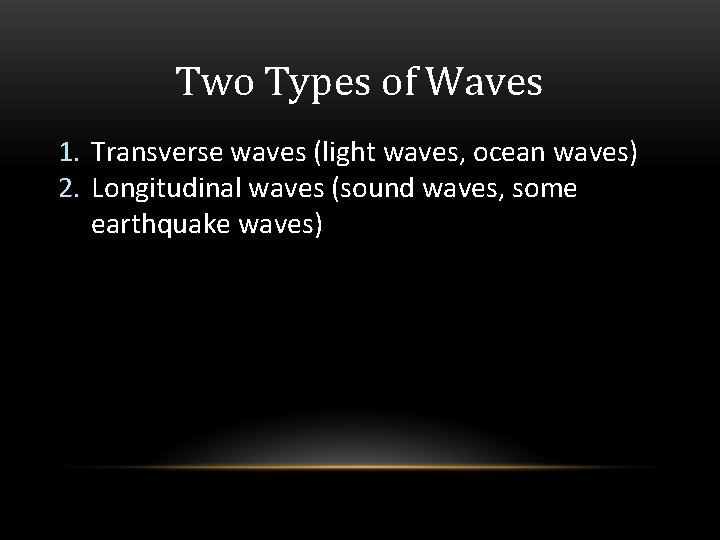Two Types of Waves 1. Transverse waves (light waves, ocean waves) 2. Longitudinal waves (sound waves, some earthquake waves)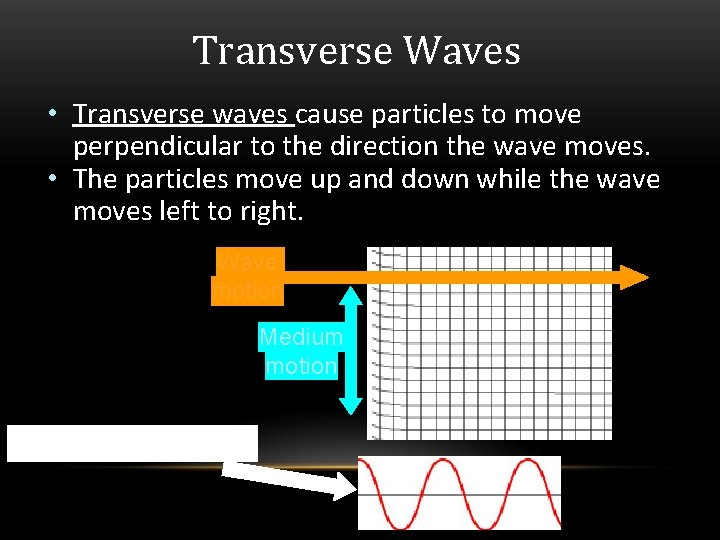Transverse Waves • Transverse waves cause particles to move perpendicular to the direction the wave moves. • The particles move up and down while the wave moves left to right. Wave motion Medium motion Looks Like This!!!!!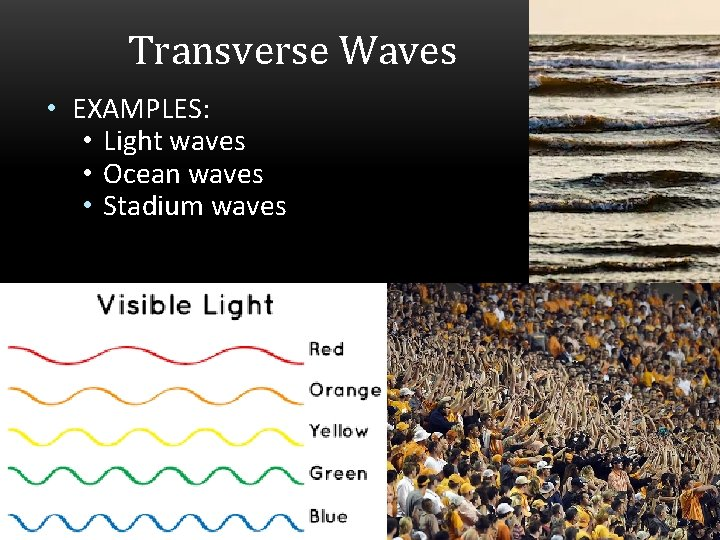Transverse Waves • EXAMPLES: • Light waves • Ocean waves • Stadium waves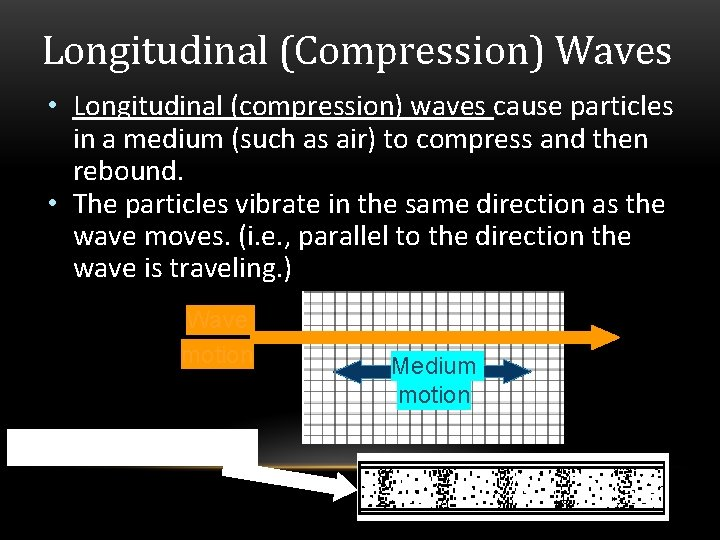Longitudinal (Compression) Waves • Longitudinal (compression) waves cause particles in a medium (such as air) to compress and then rebound. • The particles vibrate in the same direction as the wave moves. (i. e. , parallel to the direction the wave is traveling. ) Wave motion Looks Like This!!!!! Medium motion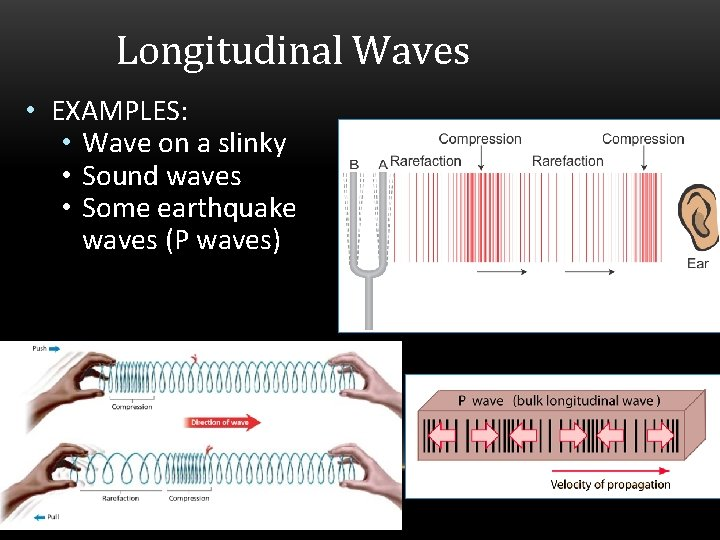Longitudinal Waves • EXAMPLES: • Wave on a slinky • Sound waves • Some earthquake waves (P waves)PART 3: LIGHT WAVES (Example of a Transverse Wave)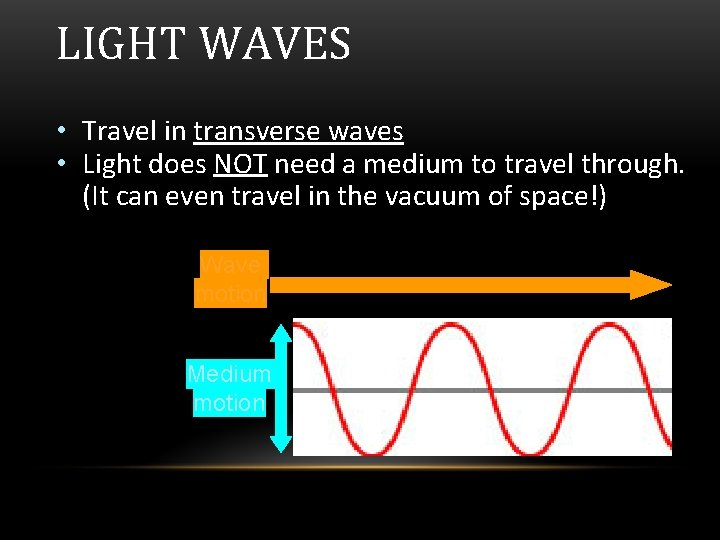LIGHT WAVES • Travel in transverse waves • Light does NOT need a medium to travel through. (It can even travel in the vacuum of space!) Wave motion Medium motion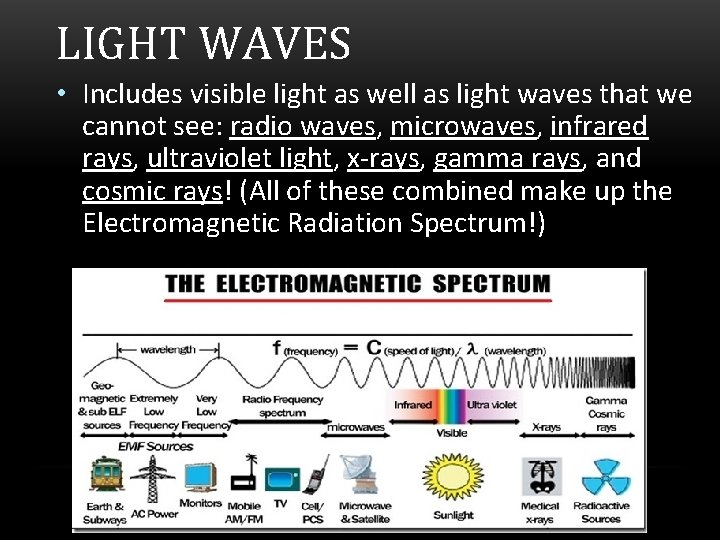LIGHT WAVES • Includes visible light as well as light waves that we cannot see: radio waves, microwaves, infrared rays, ultraviolet light, x-rays, gamma rays, and cosmic rays! (All of these combined make up the Electromagnetic Radiation Spectrum!)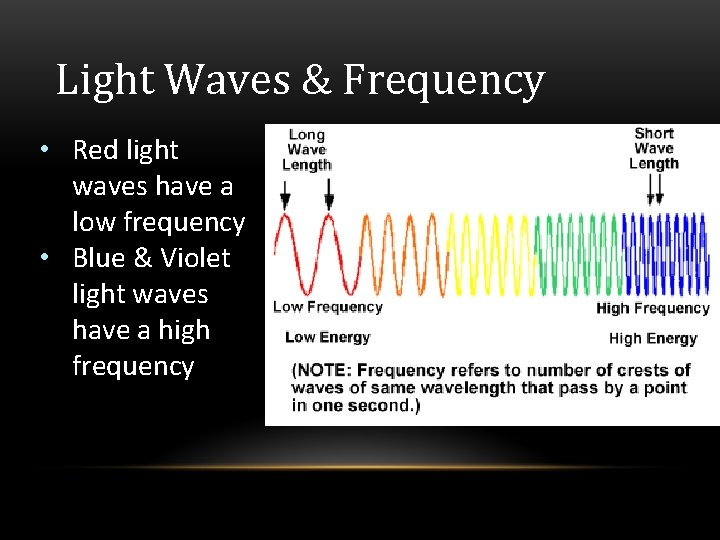Light Waves & Frequency • Red light waves have a low frequency • Blue & Violet light waves have a high frequency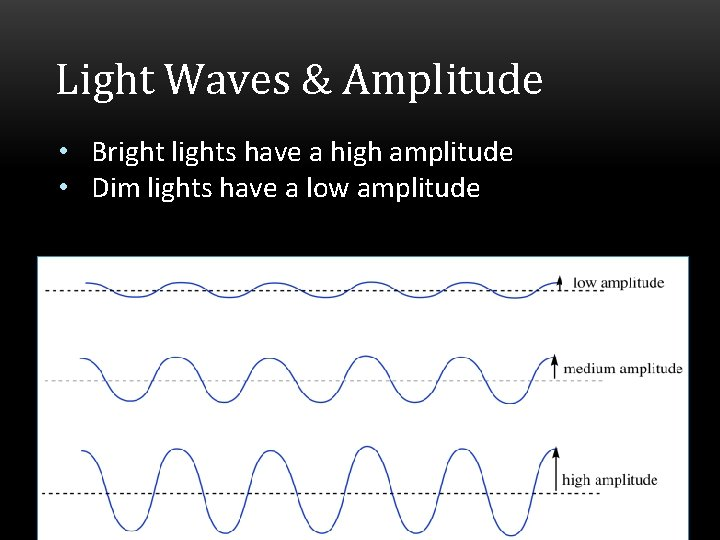Light Waves & Amplitude • Bright lights have a high amplitude • Dim lights have a low amplitudePART 4: SOUND WAVES (Example of a Longitudinal Wave)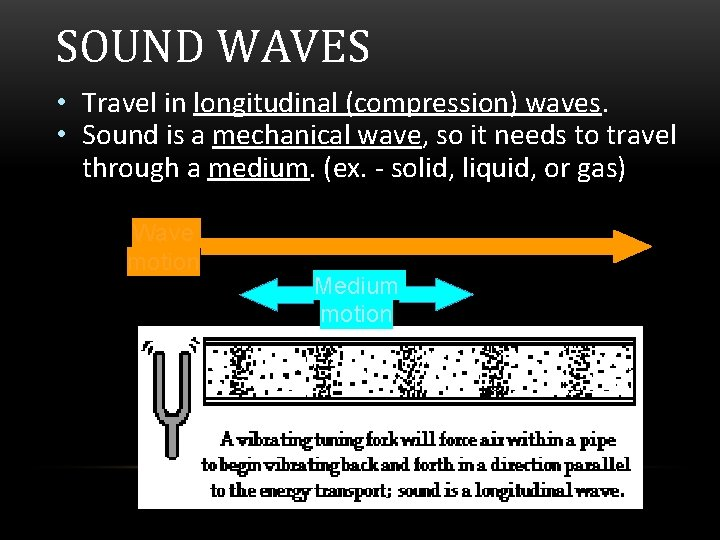SOUND WAVES • Travel in longitudinal (compression) waves. • Sound is a mechanical wave, so it needs to travel through a medium. (ex. - solid, liquid, or gas) Wave motion Medium motionSound Waves & Frequency • High pitch sound waves have a high frequency • Low pitch sound waves have a low frequency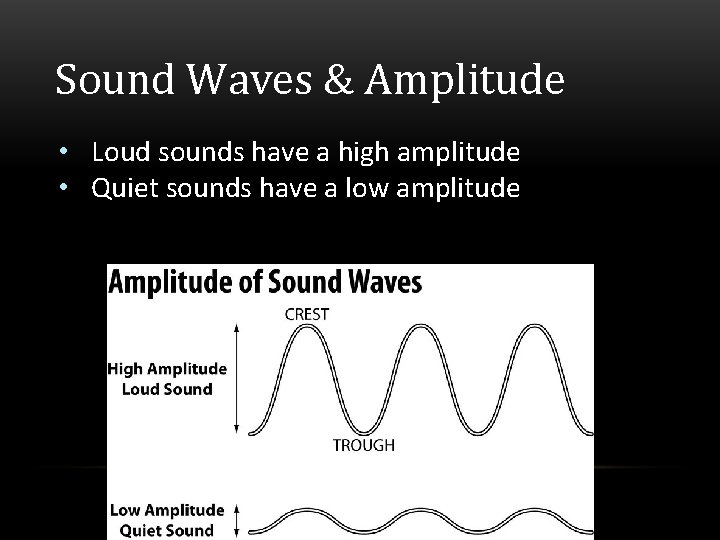Sound Waves & Amplitude • Loud sounds have a high amplitude • Quiet sounds have a low amplitudePART 5: ENERGY OF WAVES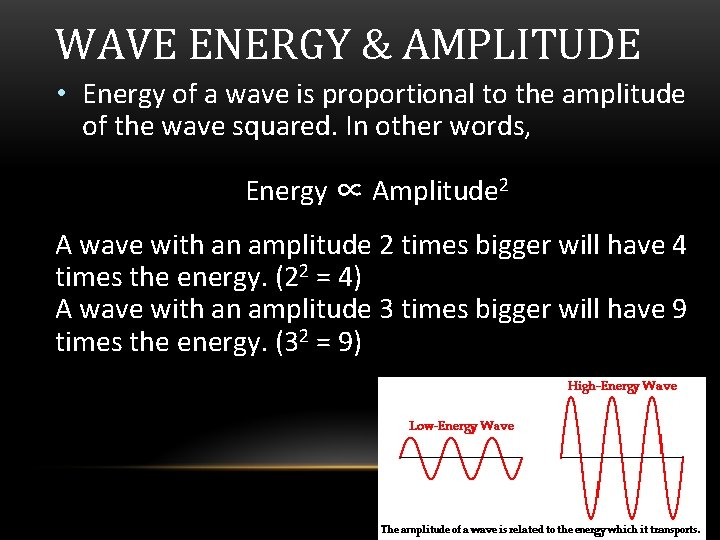WAVE ENERGY & AMPLITUDE • Energy of a wave is proportional to the amplitude of the wave squared. In other words, Energy ∝ Amplitude 2 A wave with an amplitude 2 times bigger will have 4 times the energy. (22 = 4) A wave with an amplitude 3 times bigger will have 9 times the energy. (32 = 9)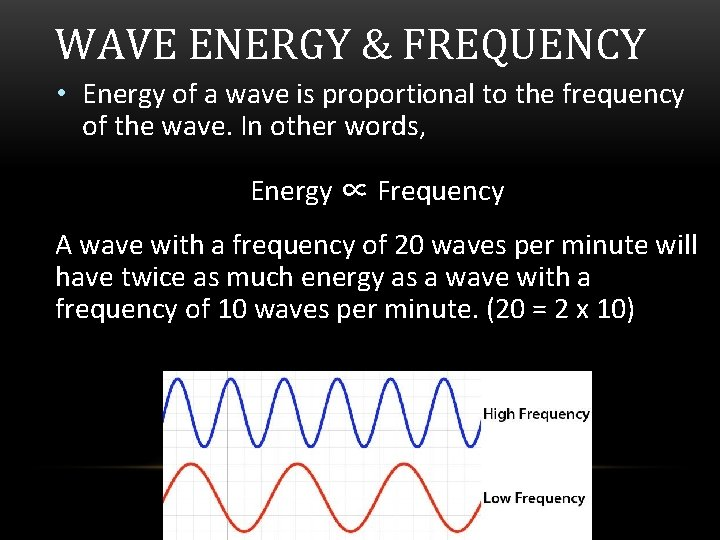WAVE ENERGY & FREQUENCY • Energy of a wave is proportional to the frequency of the wave. In other words, Energy ∝ Frequency A wave with a frequency of 20 waves per minute will have twice as much energy as a wave with a frequency of 10 waves per minute. (20 = 2 x 10)